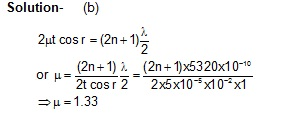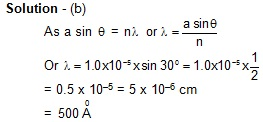# Questions and Solutions part 3

1. When light is incident on a soap film of thickness 5 × 10–5 cm, wavelength reflected maximum in the visible region is 5320 Å. Refractive index of the film will be
(a) 1.22    (b) 1.33    (c) 1.51        (d) 1.83.2. A ray of unpolarised light is incident on a glass plate of refractive index 1.54 at polarising angle, then angle of refraction is
(a) 33    (b) 44    (c) 57        (d) 903. Two waves of same intensity produce interference. If intensity at maximum is 4I, then intensity at the minimum will be
(a) 0         (b) 2I
(c) 3I        (d) 4I.

Solution - (a)
I1 = I2 = I = a2
Imax. = (a + a)2 + (2a)2 = 4a2 = 4I
Imin. = (a - a)2 = 0

4. First diffraction minima due to a single slit of width 1.0 x 10–5 cm is at 300. Then wavelength of light used is

a. 400 Å   b.   500Å    c.   600Å    d.    700Å5. Light of wavelength   is normally incident on a slit. Angular position of second minimum from central maximum us 300. Width of the slit should be
(a) 12 x 10–5 cm        (b) 18 x 10–5 cm
(c) 24 x 10–5 cm        (d) 36 x 10–5 cm6. A slit 5 cm wide when irradiated by waves of wavelength 10 mm results in the angular spread of the central maxima on either side of incident light by about

Solution - (d)
Angular spread on either side is given by
θ = λ/a = λ/5 radians

7. In Young's double slit experiment, ten slits separated by a distance of 1 mm are illuminated by a monochromatic light source of wavelength 5 x 10–7 m. If the distance between slit and screen is 2 metre, then separation of bright lines in the interference pattern will be
(a) 0.5 mm        (b) 1.0 mm
(c) 1.5 mm        (d) 1.75 mm